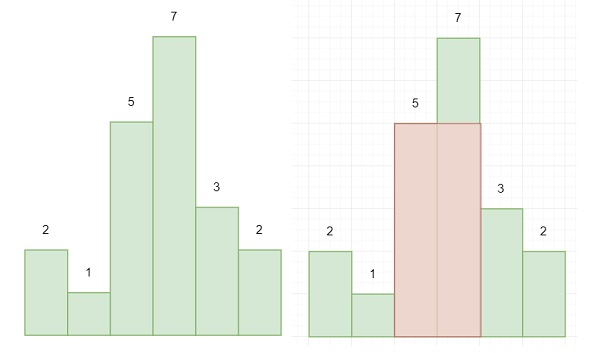# Largest Rectangle in Histogram in Python

Suppose we have one integer array that is representing the height of a histogram. Each bar has unit width. We have to find the largest area rectangle as follows −To solve this, we will follow these steps −

• Create stack, set i := 0, ans := 0

• while i < size of heights, then

• if stack has 0 elements or height of stack top element <= height[i], then

• insert i into stack, increase i by 1

• otherwise −

• x := stack top element, delete from stack.

• height := heights[x]

• temp := height * (i * stack[-1] – 1) when stack is not empty otherwise temp := height * i

• ans := max of ans and temp

• while stack is not empty −

• x := stack top element

• height := height[x], delete from stack

• temp := height * length of heights – stack top element – 1 when stack is not empty, otherwise temp := length of heights

• ans := max of ans and temp

• return ans

## Example

Let us see the following implementation to get a better understanding −

Live Demo

class Solution(object):
def largestRectangleArea(self, heights):
stack = []
i = 0
ans = 0
while i < len(heights):
if len(stack) == 0 or heights[stack[-1]]<=heights[i]:
stack.append(i)
i+=1
else:
x = stack[-1]
stack.pop()
height = heights[x]
temp = height * (i-stack[-1]-1) if len(stack)!= 0 else height * i
ans = max(ans,temp)
while len(stack)>0:
x = stack[-1]
height = heights[x]
stack.pop()
temp = height * (len(heights)-stack[-1]-1) if len(stack)!= 0 else height* len(heights)
ans = max(ans,temp)
return ans
ob = Solution()
print(ob.largestRectangleArea([2,1,5,7,3,2]))

## Input

[2,1,5,7,3,2]

## Output

12